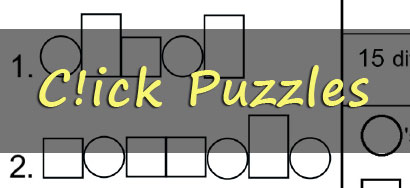## Other Puzzles## Numbergrid Puzzles

Uncover the hidden pattern in the grid using pure logic based solely on the numbers given at the start of every row and column. Our Numbergrid puzzles range in size from 5x5 squares to 25x25 squares. Click here for puzzles »## Balance Quest Puzzles

Complete each puzzle by filling in the empty boxes with positive and negative numbers in a way that balances the sums between all values in the grid, without reusing any number in the white or grey columns. Click here for puzzles »## Logicross Puzzles

Use each given letter or letter-grouping once, and only once, in the appropriate numbered rows and columns in the grid to uncover a hidden quote! Use only pure logic to figure out where each letter group should be placed - no other knowledge is required! Click here for puzzles »## Mathdoku Puzzles

Mathdoku puzzles are a mix between a math puzzle and a Sudoku puzzle. You must enter the numbers 1 through 9 into each empty white square, using each number exactly once, so that all six mathematical equations on the grid are fulfilled. Click here for puzzles »## C!ick Puzzles

Use the given shapes and patterns to reveal a themed list of words or phrases! A new C!ick puzzle will be published each week. Click here for puzzles »## PhuTAri Puzzles

Distribute all of the numbers among the cells so that the product of all of the numbers in each cell are all the same. Click here for puzzles »